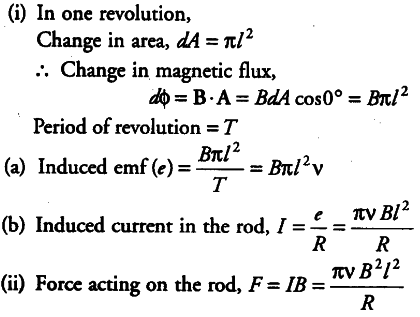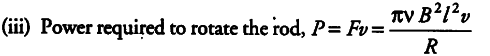# A metallic rod of length l and resistance R is rotated with a frequency v

A metallic rod of length l and resistance R is rotated with a frequency v, with one end hinged at the centre and the other end at the circumference of a circular metallic ring of radius l, about an axis passing through the centre and perpendicular to the plane of the ring. A constant and uniform magnetic field B parallel to the axis is present everywhere.
(i) Derive the expression for the induced emf and the current in the rod.
(ii) Due to the presence of the current in the rod and of the magnetic field, find the expression for the magnitude and direction of the force acting on this rod.
(iii) Hence, obtain the expression for the power required to rotate the rod.The external force required to rotate the rod opposes the Lorentz force acting on the rod/external force acts in the direction opposite to the Lorentz force.